A small-signal voltage V(t)=Vo sinωt is applied across an ideal capacitor C
(a) over a full cycle, the capacitor C does not consume any energy from the voltage         source
(b) current I(t) is in phase with voltage V(t)
(c) current I(t) leads voltage V(t) by 180°
(d) current I(t) lags voltage V(t) by 90°

Concept Questions :-

Rms and average values
High Yielding Test Series + Question Bank - NEET 2020

Difficulty Level:

An inductor 20 mH, a capacitor 50μF, and a resistor 40Ω are connected in series across a source of emf V=10sin340t. The power loss in the AC circuit is:

1. 0.67 W

2. 0.78W

3. 0.89 W

4. 0.46 W

Concept Questions :-

Different types of AC Circuits
High Yielding Test Series + Question Bank - NEET 2020

Difficulty Level:

A resistance 'R' draws power 'P' when connected to an AC source. If an inductance is now placed in series with the resistance, such that the impedance of the circuit becomes 'Z' the power drawn will be:

Concept Questions :-

Different types of AC Circuits
High Yielding Test Series + Question Bank - NEET 2020

Difficulty Level:

A series R-C circuit is connected to an alternating voltage source. Consider two situations:

1. When the capacitor is air-filled.

2. When the capacitor is mica filled.

Current through the resistor is I and voltage across the capacitor is V then

(a)Va<Vb

(b)Va>Vb

(c)ia>ib

(d)Va=Vb

High Yielding Test Series + Question Bank - NEET 2020

Difficulty Level:

A coil of self-inductance L is connected in series with a bulb B and an AC source. The brightness of the bulb decreases when

(a) frequency of the AC source is decreased

(b) the number of turns in the coil is reduced

(c) a capacitance of reactance Xc=XL is included in the same circuit

(d) an iron rod is inserted in the coil

High Yielding Test Series + Question Bank - NEET 2020

Difficulty Level:

In an electrical circuit R, L, C, and an AC voltage source are all connected in series. When L is removed from the circuit, the phase difference between the voltage and the current in the circuit is $\mathrm{\pi }/3.$ If instead, C is removed from the circuit, the phase difference is again $\mathrm{\pi }/3.$ The power factor of the circuit is

(a) 1/2

(b) 1/$\sqrt{2}$

(c) 1

(d) $\sqrt{3}/2$

High Yielding Test Series + Question Bank - NEET 2020

Difficulty Level:

The instantaneous values of alternating

current and voltages in a circuit are given

as

The average power in Watts consumed in the

circuit is

(a) $\frac{1}{4}$

(b) $\frac{\sqrt{3}}{4}$

(c) $\frac{1}{2}$

(d) $\frac{1}{8}$

High Yielding Test Series + Question Bank - NEET 2020

Difficulty Level:

In a coil of resistance 10$\mathrm{\Omega }$, the induced

current developed by changing magnetic

flux through it is shown in the figure as a

function of time. The magnitude of change

in flux through the coil in weber is-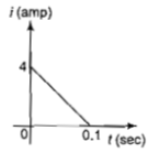(a) 8

(b) 2

(c)6

(d)4

Concept Questions :-

AC vs DC
High Yielding Test Series + Question Bank - NEET 2020

Difficulty Level:

In an AC circuit an alternating voltage  volt is connected to a capacitor  The $\mathrm{rms}$ value of the current in the circuit is

(a) 100 mA

(b) 200 mA

(c) 20 mA

(d) 10 mA

High Yielding Test Series + Question Bank - NEET 2020

Difficulty Level:

The current  in a coil varies with time as shown in the figure. The variation of induced emf with time would be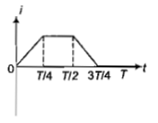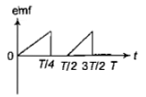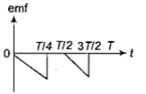(a)                                                                                         (b)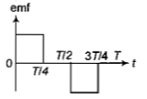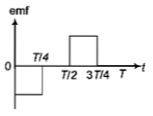(c)                                                                                             (d)